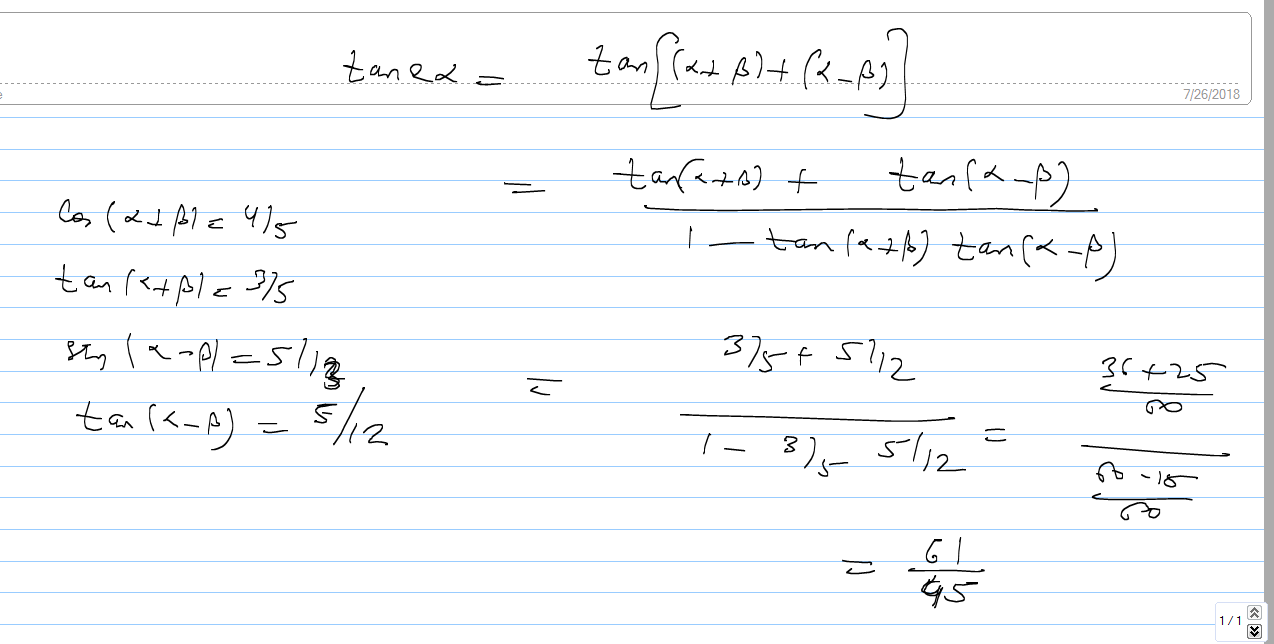×#### Thank you for registering.

One of our academic counsellors will contact you within 1 working day.

Click to Chat

1800-1023-196

+91-120-4616500

CART 0

• 0

MY CART (5)

Use Coupon: CART20 and get 20% off on all online Study Material

ITEM
DETAILS
MRP
DISCOUNT
FINAL PRICE
Total Price: Rs.

There are no items in this cart.
Continue Shopping
```
If cos (α+β) = 4/5 and sin (α-β) = 5/13 Then tan 2α =?
If cos (α+β) = 4/5 and sin (α-β) = 5/13Then tan 2α =?

```
2 years agoDeepak Kumar Shringi
4404 Points
``````
2 years ago
```							Cos(α+β)=4/5, Tan(α+β)=3/4Sin(α-β)=5/13, Tan(α-β)=5/12Tan(2α)= tan[(α+β)+(α-β)]              =[tan(α+β)+tan(α-β)]/1-tan(α+β)tan(α-β)              =(3/4 + 5/12)/1-(3/4)(5/12)              =[(36+20)/48]/[(48-15)/48]              =56/33
```
2 years agoDeepak Kumar Shringi
4404 Points
```							by mistake i have taken tan alpha+beta as ¾ but the method is same
```
2 years ago
```							 Cos(α+β)=4/5, Tan(α+β)=3/4Sin(α-β)=5/13, Tan(α-β)=5/12Tan(2α)= tan[(α+β)+(α-β)]              =[tan(α+β)+tan(α-β)]/1-tan(α+β)tan(α-β)              =(3/4 + 5/12)/1-(3/4)(5/12)              =[(36+20)/48]/[(48-15)/48]              =56/33
```
2 years ago
Think You Can Provide A Better Answer ?

## Other Related Questions on Trigonometry

View all Questions »### Course Features

• 731 Video Lectures
• Revision Notes
• Previous Year Papers
• Mind Map
• Study Planner
• NCERT Solutions
• Discussion Forum
• Test paper with Video Solution### Course Features

• 31 Video Lectures
• Revision Notes
• Test paper with Video Solution
• Mind Map
• Study Planner
• NCERT Solutions
• Discussion Forum
• Previous Year Exam Questions